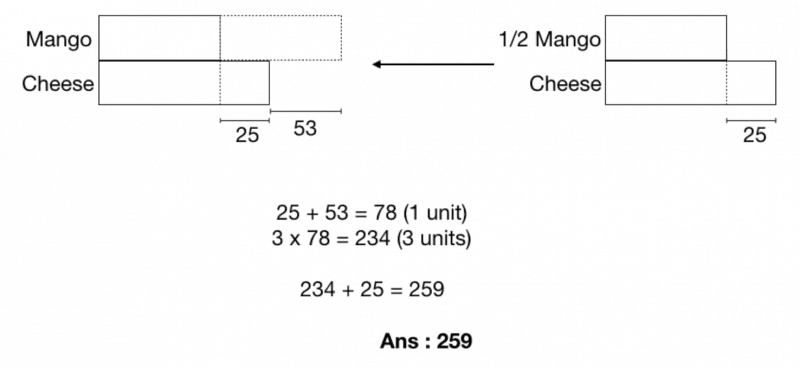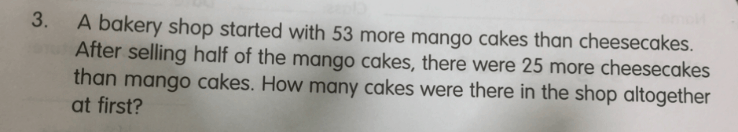# QuestionMost of the time when the question give you the Difference between 2 objects/person at first, you can make use of the model strategy and work forward. Doing so in this case will result in a decimal (1/2 x 53 = 26.5) which is not wrong, you can still get the correct answer in the end.

However, if your child is not comfortable with decimals, he can explore the option of working the model backwards as shown in my diagram. Hence, the total number of mango and cheese cakes at first is 259

0 Replies 0 LikesWhat I know?

Mango= 53 more than cheesecake

Mango- half … 25 more cheesecakes than mango

At first= ?

(It would be easier if you use ratio to solve this qn)

• Try using guess and check method
• Try  drawing a model

mango- u

u + 53

Mango                                 Cheesecake

s           u+53                                            u

p              – half                               no change

e                  u+ 24.5                               u+25

that is using the state,process,end method. (SPE)

now: 2u + 24.5+ 25= 2u+ 49.5

u= 49.5 divide by 2

= 24.75

at first= 24.75 + 53+ 24.73

= 102.50

ans: 102.50

rounded= 103…

I am not vv sure that my ans is correct as im not using the ratio method as I do not think that you have learnt ratio yet…

0 Replies 1 Like